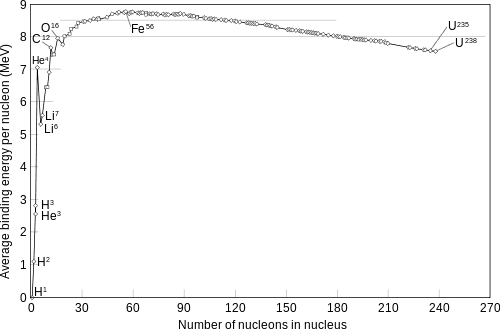# Binding energy and favorable reactions

letec
[Moderator note: Thread moved from technical section, thus no template is shown]

Hi everyone!
I'm currently learning nuclear physics and I have a question:
Watching the binding energy per nucleon curve, is the following reaction energetically favorable?
2H + 4He -> 6Li
And why?Thanks!!!

Mentor
Why don't you take the values from the diagram and check for yourself? Better (more accurate): Look up the binding energies (or the masses) and calculate it.

letec
Why don't you take the values from the diagram and check for yourself? Better (more accurate): Look up the binding energies (or the masses) and calculate it.

Mentor
I moved you to the homework section.

Well, then use the diagram and check it.

letec
I moved you to the homework section.

Well, then use the diagram and check it.
That's exactly my question... The binding energy of the 4He is bigger than the B.E of the product. Is that it? or is there something more?

Mentor
What about the binding energy of deuterium?

letec
What about the binding energy of deuterium?
well it's smaller! and that is my question!

Mentor
No, that is the homework question.

What is the combined binding energy of D+4He?

letec
No, that is the homework question.

What is the combined binding energy of D+4He?
do you mean for 2H + 4He -> 6Li:
2*1 MeV + 4*7MeV = 30MeV < 6*5.3 = 31.8 therefore the reaction is energetically favorable?

Mentor
Sure.

To conserve energy and momentum, you would need an additional photon in the final state.

letec
Sure.

To conserve energy and momentum, you would need an additional photon in the final state.
Thanks for the help! :)